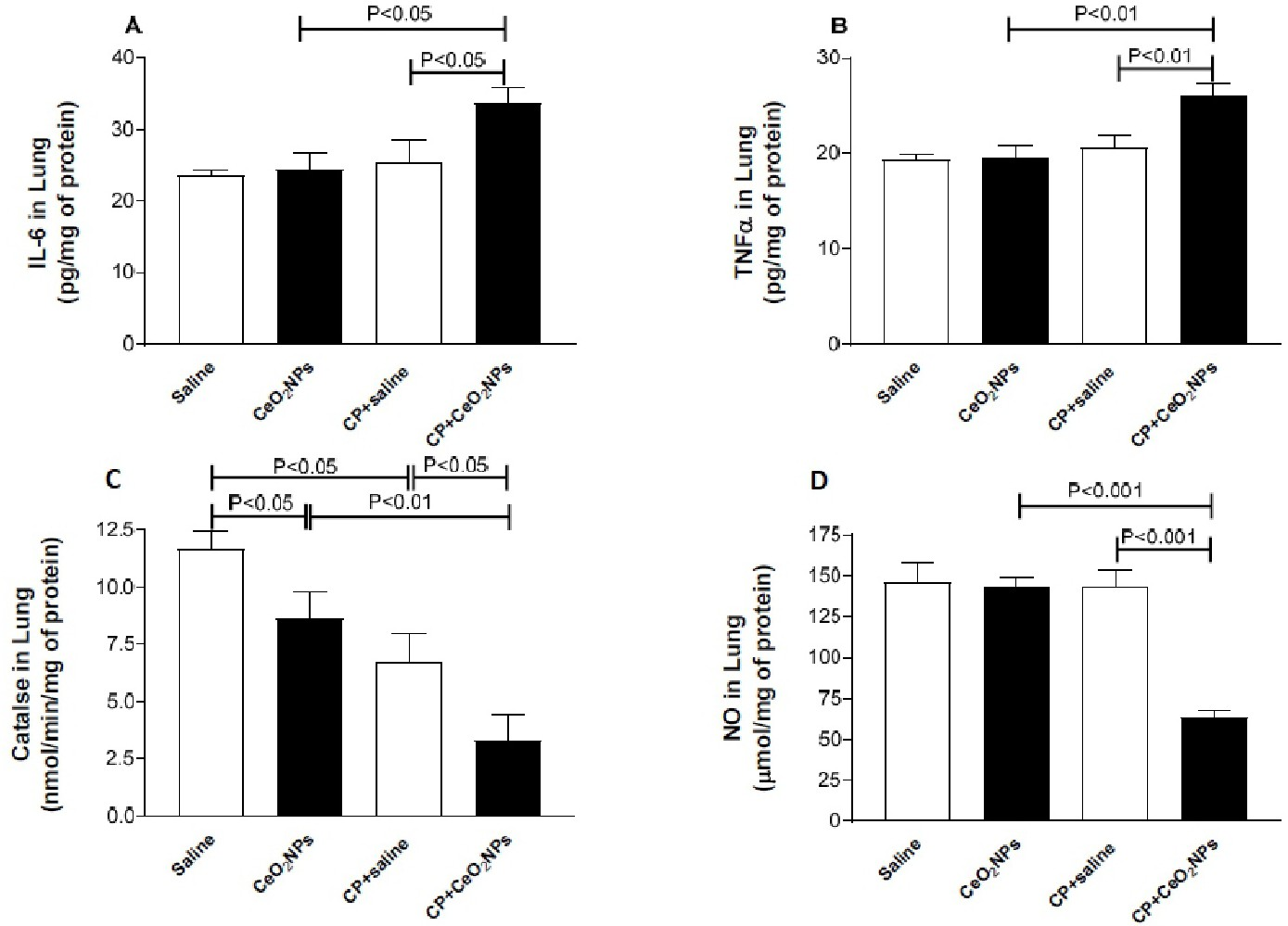Fig. 7. interleukin 6 (IL-6, A), tumor necrosis factor α (TNFα, B), catalase (C) and total nitric oxide (NO, D) levels in lung homogenates of Wistar rats intratracheally instilled with either saline (control) or cerium oxide nanoparticles (CeO2 NPs) with or without cisplatin (CP) administration. Data are mean ± SEM. IL-6: saline (n=6), CeO2 NPs (n=6), CP+saline (n=6) and CP+ CeO2 NPs (n=6). TNFα: saline (n=7), CeO2 NPs (n=7), CP+saline (n=6) and CP+ CeO2 NPs (n=6). Catalase: saline (n=6), CeO2 NPs (n=5), CP+saline (n=5) and CP+ CeO2 NPs (n=5). NO: saline (n=8), CeO2 NPs (n=8), CP+saline (n=8) and CP+ CeO2 NPs (n=8).